×
INTELLIGENT WORK FORUMS
FOR ENGINEERING PROFESSIONALS

Are you an
Engineering professional?
Join Eng-Tips Forums!
• Talk With Other Members
• Be Notified Of Responses
• Keyword Search
Favorite Forums
• Automated Signatures
• Best Of All, It's Free!

*Eng-Tips's functionality depends on members receiving e-mail. By joining you are opting in to receive e-mail.

#### Posting Guidelines

Promoting, selling, recruiting, coursework and thesis posting is forbidden.

# phase shift calculation

## phase shift calculation

(OP)
I have a sine wave plotted, i.e. y = sin(x)

I have a second wave plotted, where y = sin(2x)+1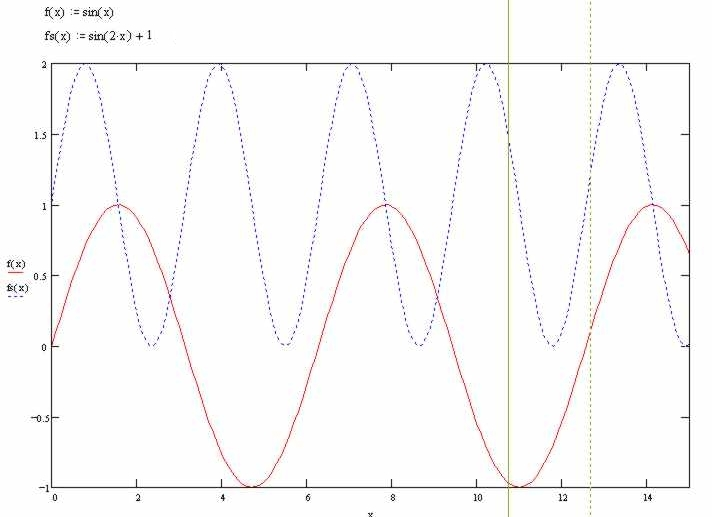I now wish to introduce a phase shift, such that the latter wave becomes tangential with the former.

Whether the shift is in the X+ or X- direction is irrelevant to me.

Can anyone please explain how to calculate this phase shift, and illustrate it with an Excel graph, to show that the results of the calculation actually do provide the correct phase shift to create the condition where the waves are actually tangential at certain periodic instants.

Thanks

### RE: phase shift calculation

With different frequencies you will not get a phase shift. You will get the sum and the difference of the two original frequencies.

Bill
--------------------
"Why not the best?"
Jimmy Carter

### RE: phase shift calculation

(OP)
Sorry but what you say just isn't true.

The two curves have a frequency ratio of 1:2, so they will be tangent on a regular basis at a certain phase shift.

I have already done it by iteration and proved it.

I just want a more methodical solution other than iteration.

### RE: phase shift calculation

Is this really a design problem? And that other problem of yours - was that also related to circuit design?

If this is your bread and butter, I think that you should earn it yourself.

If it is for school...

Gunnar Englund
www.gke.org
--------------------------------------
Half full - Half empty? I don't mind. It's what in it that counts.

### RE: phase shift calculation

(OP)
@ Skogsgurra

That was really useful.

Thanks

Why waste your time even commenting.

Do you think your comment served any purpose at all.

I know I don't.

What are you, the forum policeman or something.

### RE: phase shift calculation

I agree with Bill (waross), but using the terms as you've defined, this seems to be perfectly trivial.

Hint: Linear interpolation along the X axis with a period of, ah, the period.

Which puts the emphasis back on Gunnar's question.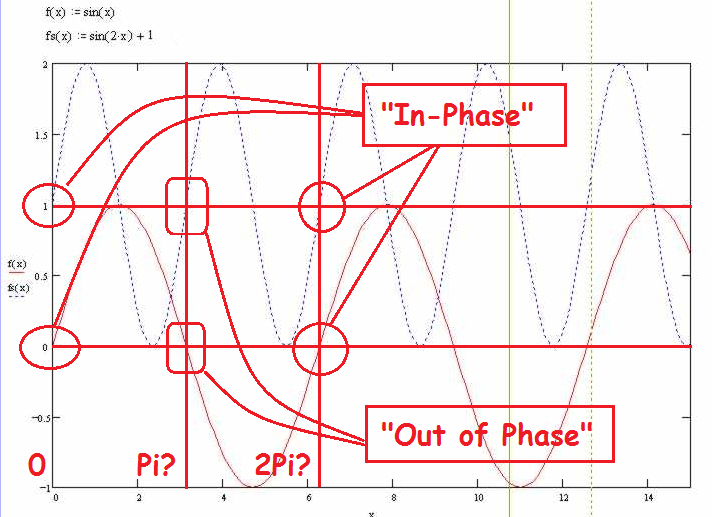### RE: phase shift calculation

This site has quite a few forum policemen.

Guidelines are listed just below the post entry box.

Promoting, selling, recruiting, coursework and thesis posting is forbidden. - Posting Guidelines

### RE: phase shift calculation

"...points of tangency..."

Ah. So trivial calculus or apply 90° phase shift (same thing).

### RE: phase shift calculation

In the electrical fora, the term "phase shift" is most almost exclusively applied to waveforms of the same frequency.
Forgive us our confusion.
Our practical applications are too much influenced by the possible changing amplitudes of one or both waveforms to ever worry about points of tangency.
How does this apply to circuit design?

Bill
--------------------
"Why not the best?"
Jimmy Carter

### RE: phase shift calculation

(OP)
I give up.

It appears I cannot explain the problem well enough.

Thanks for everyone's input.

If I find a solution I will post it here.

And just for anyone interested, the application is that I want to be able to generate arbitrary waves for my AWG, where the initial stage of the wave is defined by y = (sin(2x+s))+1, which then blends tangentially into y = sin (x)

Thanks

### RE: phase shift calculation

Good to know, at last, what the application is. If I get it right, you need to generate a waveform where one sine exist up to a certain point where it is then replaced by another sine with the condition that there is no discontinuity in the first or second derivative.

Is that right? Then tell us so. Please.

Gunnar Englund
www.gke.org
--------------------------------------
Half full - Half empty? I don't mind. It's what in it that counts.

### RE: phase shift calculation

(OP)
Yes that is it exactly.

The reason I didn't want to mention the application is because posts have a habit of veering off onto a different subject.

I didn't really want to go down a rabbit trail about AWG's

I just wanted to learn the mathematics to program it.

### RE: phase shift calculation

Your math would then need to look up the next (there are an infinite number of them) inflexion point with the correct slope (positive or negative) and then start a new sinewave with the correct delay (I prefer delay over phase shift, see Bill's comment)and starting level.

It is not a very complicated math, but it may overtax the capability of your AWG at higher frequencies and running real time. I would use a PC and an external D/A for this.

Gunnar Englund
www.gke.org
--------------------------------------
Half full - Half empty? I don't mind. It's what in it that counts.

### RE: phase shift calculation

#### Quote:

I have a sine wave plotted, i.e. y = sin(x)

I have a second wave plotted, where y = sin(2x)+1

I now wish to introduce a phase shift, such that the latter wave becomes tangential with the former.
Let me give it a try
y1 = sin(x)
y2 = sin(2x + theta)+1
y1' = cos(x)
y2' = 2*cos(2x+theta)

In order to be tangent, find x and theta such that
y1 = y2 [eq1]
y1' = y2' [eq2]

plug in definitions of y1, y2, y1', y2' to give the following:
sin(x) = sin(2x + theta)+1 [eq 1a]
cos(x) = 2*cos(2x+theta) [eq 2a]

LHS(eq1a)^2 + LHS(eq2a)^2 = 1 => RHS(eq1a)^2 + RHS(eq2a)^2 = 1
(sin(2x + theta)+1)^2 + (2*cos(2x+theta))^2 = 1
[sin^2(2x + theta) + 2*sin(2x + theta) + 1] + 4 * cos^2(2x+theta) = 1

Subtract 1 from each side
sin^2(2x + theta) + 2*sin(2x + theta) + 4 * cos^2(2x+theta) = 0

Substitute 4 * cos^2(2x+theta) = 4*(1-sin^2(2x+theta))
sin^2(2x + theta) + 2*sin(2x + theta) + 4 * (1-sin^2(2x+theta)) = 0
sin^2(2x + theta) + 2*sin(2x + theta) + 4 - 4 *sin^2(2x+theta)) = 0
-3*sin^2(2x + theta) +2*sin(2x + theta) + 4 = 0

We recognize the quadratic form az^2 + b*z + c = 0 where
z = 2*sin(2x+theta)
a = -3
b = 2
c = 4

solution z = [-b +/- sqrt(b^2-4ac)]/2a = [-2 +/-sqrt(4+48)] / (-6)
z = [-2 +/- sqrt(52)] / (-6)
arbitrarily pick try the root which is positive
z = [-2 - |sqrt(52)}[ / (-6)
z = 1/3 + |sqrt(52)|/6 = 1/3 + sqrt(52/36)
z = 1/3 + |sqrt(4/3)|
z = |sqrt(7/3)|
2*sin(2x+theta) = |sqrt(7/3)|
sin(2x+theta) = 0.5 * |sqrt(7/3)|
There are two values of the argument (2x+theta) between 0 and Pi to satisfy above.
Of course also repeating solutions separated by n*2pi

And there is another set of solutions if you pick the -sqrt
I'm not going to go there because I may have made some algebra errors already.
I leave it to whoever wants to check it...

=====================================
(2B)+(2B)' ?

### RE: phase shift calculation

#### Quote (electricpete)

z = 1/3 + |sqrt(4/3)|
z = |sqrt(7/3)|
It looks right to me up to the first line.
The 2nd line is wrong. Edit seems broken.

=====================================
(2B)+(2B)' ?

### RE: phase shift calculation

Apologies that my previous attempts went off on a tangent... Trying to understand the question is often where I get confused.

Let's try again.

For 2. y = sin(2x)+1, the +1 offset has zero effect on the slope, so the +1 can simply be dropped (ignored).

For any y = sin(...), the slope (or tangent) is the derivative, which is just y = cos(...).

This derivative of any sin being cos can be done practically by noting that sin and cos are exactly the same thing, with a horizontal relative 90° phase shift (the period of each signal).

The sin wave becomes its own slope by simply kicking it sideways by 90°.

Combining the two (eliminating the unnecessary +1 vertical offset and perhaps sliding each waveform horizontally each by their own 90° to each become their own derivative) then the two new curves will obviously cross where their slopes are equal.

This answers the question to know where the slopes of the original waveform are equal.

Measuring the relative 'phase' (*) at other points is a simple linear measurement (horizontally) with a ruler, to the nearest matching point (remember that amplitude = slope).

( * The vocabulary gets a bit twisted due to the point originally raised by Bill (waross). But I think that the last step here is obvious at this point.)

I hope this helps.

### RE: phase shift calculation

I don't believe that the offset may be ignored. The OP is not just looking for equal slope but equal slope and equal magnitude. If the waveforms are shifted so that the peaks align then the slopes are equal but due to the offset, the waveforms are not tangent.
I don't believe that this may be solved without including the instantaneous magnitude.
Any change in either the peak to peak value of either waveform or the vertical offset between the waveforms will shift the point of tangency.
It is difficult to visualize a real world application. Given the effect of circuit loading on the magnitude of waveforms, it may be difficult to maintain exactly equal magnitudes in the real world.
Remember also that as the frequency changes, the impedance of any but a purely resistive load circuit also changes. That will change the offset between the EMF and the current waveforms. Or as, we use the term, the "Phase shift" between the EMF and the current of each signal will change.

Bill
--------------------
"Why not the best?"
Jimmy Carter

### RE: phase shift calculation

Respectfully,

#### Quote (VE1BLL)

(remember that amplitude = slope).
If you change the offset, you change the absolute magnitude.

Bill
--------------------
"Why not the best?"
Jimmy Carter

### RE: phase shift calculation

"...equal slope and equal magnitude."

The method that I described will, I think, reveal the points in time where slopes are equal. I'd expect perhaps two per repeating cycle.

The original waveforms are equal in amplitude where they trivially intersect, obviously two points per repeating cycle.

Clearly the 'equal phase' two points in time are not the same as the 'equal amplitude' two points in time.

Given two different signals, in order for the amplitudes to be equal, the waveforms must cross over. Obviously the slopes must be different at that same point !!### RE: phase shift calculation

"...amplitude = slope..."

Amplitude of the derivative waveform (cos, or shifted sin) represents the slope of the original (sin).

If one wishes to leave the +1 offset in Equation 2, that's fine. That +1 offset will still disappear when the derivative is taken anyway.

### RE: phase shift calculation

Darn Post Edit function isn't working.

Typo.

Clearly the 'equal slope' two points in time are not the same as the 'equal amplitude' two points in time.

### RE: phase shift calculation

OK, now that I understand what you're trying to do, the solution presented by VE1BLL from Wolfram Alpha in your other thread was almost the correct answer. This is one reason why you should try to keep variants of a topic together, since it turns out that your only issue with the other thread was that the second equation did not reflect the derivative correctly, and had you presented the actual problem, instead of your attempted solution, this thread would have been completely unnecessary, as the error would have been immediately revealed.

sin(x) = sin(2x+s)+1
This is the equation for having the two curves intersect, where s is the phase required to get the intersection and matching slope

cos(x) = 2cos(2x+s) instead of cos(x) = cos(2x+s)
This is the equation for having the same slope at the point of the intersection

The corrected Wolfram Alpha link is below. Unfortunately, those nice neat solutions from last time didn't appear this time; they're substantially more complex.
https://www.wolframalpha.com/input/?i=Find+x+and+s...)

TTFN (ta ta for now)
I can do absolutely anything. I'm an expert! https://www.youtube.com/watch?v=BKorP55Aqvg
FAQ731-376: Eng-Tips.com Forum Policies forum1529: Translation Assistance for Engineers

### RE: phase shift calculation

The trailing bracket escaped from the link.### RE: phase shift calculation

#### Quote:

Given two different signals, in order for the amplitudes to be equal, the waveforms must cross over. Obviously the slopes must be different at that same point !!
I'm not sure about the 2nd statement.

But more important I think the first statement is incorrect, and examining this behavior helps us graphically envisioning the solution to op's problem.

As a simple example look at two curves: cos(pi*t) and cos(2*pi*t)
Examine behavior at t=0.
They are not crossing over.
Both they have the same value (1) and the same slope (0).

It is similar situation for the op's problem. It is not a point of crossing but a point of contact/tangency between the two curves.

In the way it is plotted in original post the upper lobe of the lower curve intersets with the lower lobe of the upper graph at two points (roughly x=0.9 and x=2.8). If we slide the upper curve to the right gradually these twp points of intersection will move. If we slide far enough to the lower lobe will not contact the upper lobe at all and we have zero points of intersection.

Obviously somewhere between the two points of intersection and zero points of intersection, we have to pass through a place where there is only one point of intersection of the two curves. And if they do not cross but have one point of intersection, then we conclude that will be a point of tangency. I think you can apply similar logic in any region of two "smooth" curves where one is concave up and the other concave down.

Attached graph illustrates. Blue and red curves are the op's curves. Shift the Red curve to the right and it turns into the red curve and those two points of contact merge into one local point of contact (if you shifted any further they would turn into zero local points of contact).

=====================================
(2B)+(2B)' ?

### RE: phase shift calculation

#### Quote:

I think you can apply similar logic in any region of two "smooth" curves where one is concave up and the other concave down.
If I could edit, I'd get rid of that because it doesn't encompass my simple example of the two curves: cos(pi*t) and cos(2*pi*t).

=====================================
(2B)+(2B)' ?

### RE: phase shift calculation

#### Quote (electricpete correction)

Attached graph illustrates. Blue and red curves are the op's curves. Shift the Red curve to the right and it turns into the red grey curve and those two points of contact merge into one local point of contact (if you shifted any further they would turn into zero local points of contact).

=====================================
(2B)+(2B)' ?

### RE: phase shift calculation

The observation was specifically in response to Bill's "...The OP is....looking for....equal slope and equal magnitude...", and in the context of the OP's two waveforms.

Sorry for the confusion. I should have included additional verbiage to put limits on the wording.

Carry on.### RE: phase shift calculation

(OP)
This isn't the optimum solution, but I'm happy to settle for this.

It is just a case of finding the minimum value of the equation shown, then the shift required is read directly from the y axis.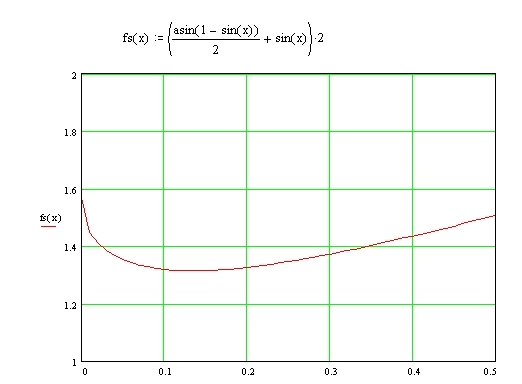Just for reference, I did also notice that I made a mistake in the very first post of the original thread where this theme began.

I incorrectly calculated the derivative of sin(2x+s)+1 as cos(2x+s), when it ought to have been 2cos(2x+s)

### RE: phase shift calculation

I guess that the phase shift is in radians. If so, is it really plausible to add arcsine (range +-Pi) and sine (range +/-1)? To me, it is a screaming unit incompatibility. Did you test this? With meaningful results?

I guess that I will be hooking up a dual channel AWG and a scope with math capability to verify all the different proposed solutions. Nothing beats reality.

But, even if I really wanted to do that, time is lacking. Hope to do it in a week or two.

Gunnar Englund
www.gke.org
--------------------------------------
Half full - Half empty? I don't mind. It's what in it that counts.

### RE: phase shift calculation

Attached xlsx file graphically shows a solution in the neighborhood (x = 3.0, theta = -1.85)
It is similar to my previous-posted pdf, except there is a correction in the curve labeling and some format changes.

Op's solution suggests theta = 1.3. If I plug in theta = 1.3 it doesn't suggest a solution, but if I plug in -1.3 into my spreadsheet (cell B2), then I see another solution around x=0.2. Maybe that is consistent with op's solution posted above if we have defined theta with opposite polarities of each other. Although I didn't follow the logic of how the op came up with his graph.

I'm not so sure about my previous analytical solution leading to z = 1/3 +/- |sqrt(4/3)| = 2*sin(2x+theta). Doesn't seem to match my graphical solutions.

=====================================
(2B)+(2B)' ?

### RE: phase shift calculation

Here is a slightly better spreadsheet... it has the x axis adjusted to show a full range 0..2*Pi (for any value of theta that gives a solution, a solution should appear in this range).

I think the solutions are in the neighborhood
theta = -1.85, x=3+n*2*pi
theta = -1.3, x=0.2+n*2*pi

=====================================
(2B)+(2B)' ?

### RE: phase shift calculation

(OP)
Here are the solutions for the case I am interested in.

There are other solutions, but this is the one I want.

Ignore the fact that the value of the shift is positive, it just refers to the shift of the other wave.

I know this works.

I've graphed it and it is perfect, as it is also in the AWG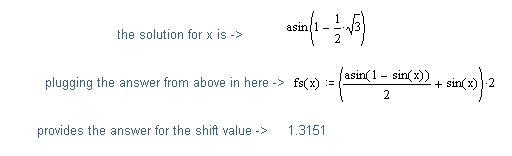### RE: phase shift calculation

since you already have Mathcad, this is the way I would do it.
http://files.engineering.com/getfile.aspx?folder=d...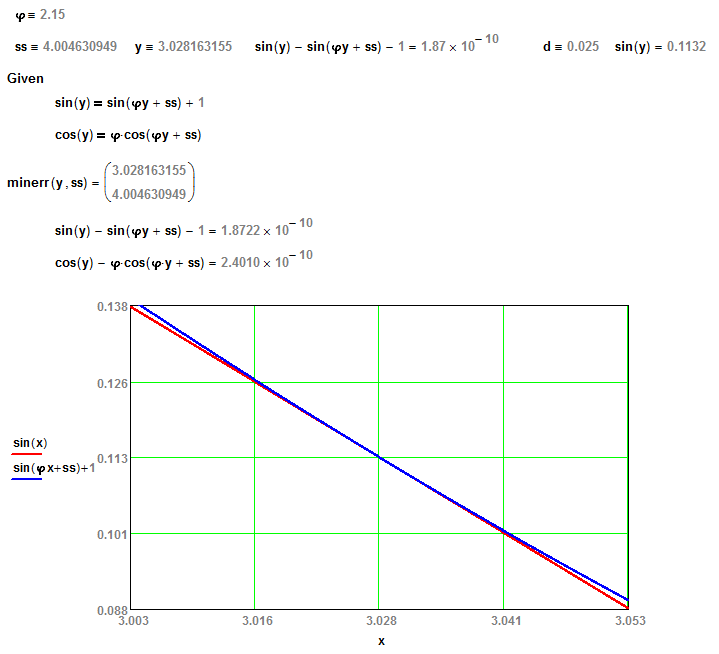TTFN (ta ta for now)
I can do absolutely anything. I'm an expert! https://www.youtube.com/watch?v=BKorP55Aqvg
FAQ731-376: Eng-Tips.com Forum Policies forum1529: Translation Assistance for Engineers

### RE: phase shift calculation

(it looks correct but I'm curious how you got there)

=====================================
(2B)+(2B)' ?

### RE: phase shift calculation

I incorrectly calculated the derivative of sin(2x+s)+1 as cos(2x+s), when it ought to have been 2cos(2x+s)
I did the same thing... which likely explains why my original answer didn't work as planned.

Dan - Owner
http://www.Hi-TecDesigns.com### RE: phase shift calculation

(OP)
Which simplifies further to ->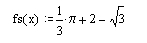### RE: phase shift calculation

MRSSPOCK, The sin(x) and sin(2x) waveforms you show remind me of the relationship between input voltage into a resistive load vs the power which the load sees (which is double the frequency of the fundamental) or, the voltage out of an inverter vs. the power delivered by that inverter (power is delivered at 2x the fundamental frequency). In either case, there is a phase shift when typical filters and other lagging or leading elements are involved. In either of these cases, the phase shift would be measured from the TOP (i.e. 90 degrees) of the fundamental, not the zero-crossing. Looking at it this way may make your trig equations easier.

Darrell Hambley P.E.
SENTEK Engineering, LLC

#### Red Flag This Post

Please let us know here why this post is inappropriate. Reasons such as off-topic, duplicates, flames, illegal, vulgar, or students posting their homework.

#### Red Flag Submitted

Thank you for helping keep Eng-Tips Forums free from inappropriate posts.
The Eng-Tips staff will check this out and take appropriate action.

#### Resources

Low-Volume Rapid Injection Molding With 3D Printed Molds
Learn methods and guidelines for using stereolithography (SLA) 3D printed molds in the injection molding process to lower costs and lead time. Discover how this hybrid manufacturing process enables on-demand mold fabrication to quickly produce small batches of thermoplastic parts. Download Now
Examine how the principles of DfAM upend many of the long-standing rules around manufacturability - allowing engineers and designers to place a partâ€™s function at the center of their design considerations. Download Now
Industry Perspective: Education and Metal 3D Printing
Metal 3D printing has rapidly emerged as a key technology in modern design and manufacturing, so itâ€™s critical educational institutions include it in their curricula to avoid leaving students at a disadvantage as they enter the workforce. Download Now

Close Box

# Join Eng-Tips® Today!

Join your peers on the Internet's largest technical engineering professional community.
It's easy to join and it's free.

Here's Why Members Love Eng-Tips Forums:

•Talk To Other Members
• Notification Of Responses To Questions
• Favorite Forums One Click Access
• Keyword Search Of All Posts, And More...

Register now while it's still free!